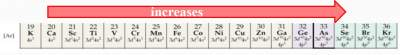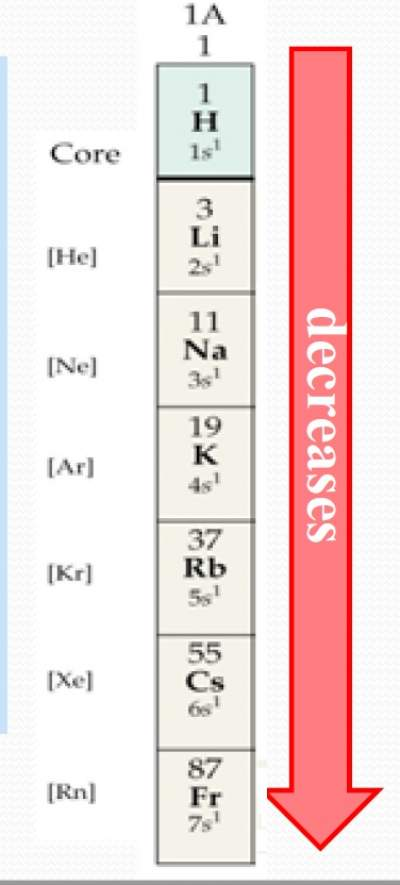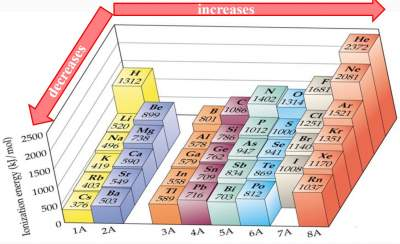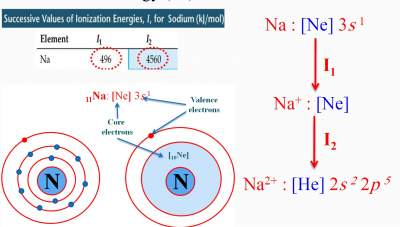# Ionization Energy (IE)

## Definition

Ionization Energy (IE) is the minimum energy required to remove an electron from the ground state of the isolated gaseous atom or ion.

The first ionization energy (I1)is the amount of energy required to remove an electron from a gaseous atom:
Na (g) → Na (g)+ + e
The second ionization energy(I2) is the energy required to remove an electron from a gaseous ion:
Na (g)+ → Na (g)2+ + e

So the question is: How does the trend of ionization energylook like in a periodic table ?

Ionization Energy increases as we move across a period.

 As we move across a period, Zeff increases. Therefore, it becomes more difficult to remove an electron, i.e., more energy is required to remove an electron from the atom (The rule of Zeff)Ionization energy decreases as we move down a group.
 As the principle quantum number (n) increases (by moving down a group), the distance of the outermost electron from the nucleus becomes larger, its attraction to the nucleus decreases, it becomes easier to remove an electron from the outermost orbitals. (The rule of n)### Exercise on Ionizing Energy versus Atomic Number

Exercise on Ionizing Energy versus Atomic Number

Check your answers here: Solution to the Exercise on Ionizing Energy versus Atomic Number### Exercise on First Ionizing Energy

Exercise 1 on First Ionizing Energy

Check your answers here: Solution to the Exercise 1 on First Ionizing Energy

Exercise 2 on First Ionizing Energy

Check your answers here: Solution to the Exercise 2 on First Ionizing Energy